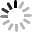Events

# What is infrared isotherm?

February 8, 2023Isotherms play an important role in the use of infrared cameras and in the analysis of infrared thermograms. Observers can intuitively observe the distribution of a certain temperature range in the infrared thermal image.

The line connecting the points with the same temperature value on the isotherm graph is called isotherm. The isotherms show different states, and the temperature represented is also different, which are divided into the following situations.

- If the isotherm is sparse, the temperature difference between different places is relatively small; if the isotherm is dense, it means that the temperature in different places is very different.

- If the isotherm is straight, it means that there are few factors affecting the temperature distribution; if the isotherm is curved, it means that there are many factors affecting the temperature distribution

- The east-west trend means that the temperature varies with latitude, and the latitude factor is the main factor; if the isotherm is parallel to the coastline, which means that the temperature varies with the distance from the sea, and the distance from the sea is the main factor.

There are several ways to interpret the isotherm:

1. Drawing method

Draw a straight line arbitrarily (representing a latitude) on the isotherm distribution map, and intersect any one of the isotherm lines, satisfying the condition of "at the same latitude". Then, take two points on the latitude line, compare the temperature of these two points, and combine the conditions to draw the conclusion.

2. Convex method

According to the convexity characteristics of the bending part of the isotherm, the high-low principle is summarized. The so-called high means that the curved part of the isotherm protrudes toward the high value direction, that is, the protruding part of each isotherm points to the direction in which the value of the isotherm increases. At this time, the curved part is a low value (relative) area. Low is the opposite of high, that is, the curved part of the isotherm protrudes toward the low value, and the curved part is the high value (relative) area. That is, "convex high is low, convex low is high".

--------------------------------------------------------------------